1. The formula for calculating moments is Moments = Force x Distance.   What is the formula for calculating Torque?
 2. What is the unit of measure for a moment?
 3a. What should the size of load A be to keep the seesaw in equilibrium? 3b. How much is the anticlockwise moment? 3c. How much is the clockwise moment?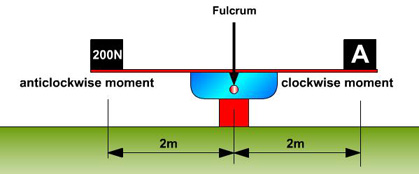4a. What should the size of load A be to keep the seesaw in equilibrium? 4b. How much is the anticlockwise moment? 4c. How much is the clockwise moment?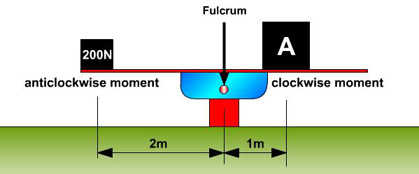5a. What should the size of load C be to keep the seesaw in equilibrium? 5b. How much is the anticlockwise moment? 5c. How much is the clockwise moment?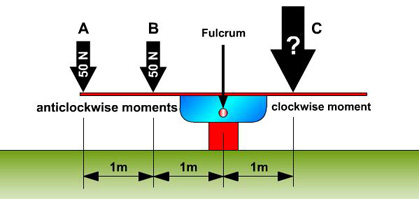6a. What should the size of load C be to keep the seesaw in equilibrium? 6b. How much is the anticlockwise moment? 6c. How much is the clockwise moment?7a. Which class of lever illustrated opposite? 7b. How much is the anticlockwise moment created by the 600N load? 7c. An effort greater than the anticlockwise moment is needed to lift the load.  What is the smallest amount of torque that will lift the load?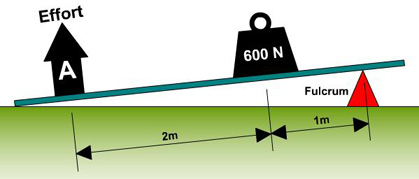8a. Which class of lever illustrated opposite? 8b. How much is the anticlockwise moment created by the 600N load? 8c. An effort greater than the anticlockwise moment is needed to lift the load.  What is the smallest amount of torque that will lift the load?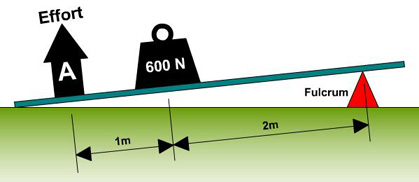9a. Which class of lever illustrated opposite? 9b. How much is the anticlockwise moment created by the 600N load? 9c. An effort greater than the anticlockwise moment is needed to lift the load.  What is the smallest amount of torque that will lift the load?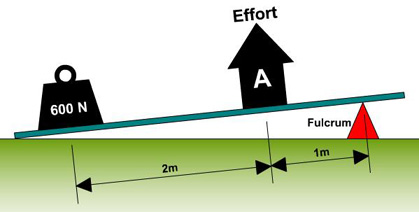10a. Which class of lever illustrated opposite? 10b. How much is the anticlockwise moment created by the 600N load? 10c. An effort greater than the anticlockwise moment is needed to lift the load.  What is the smallest amount of torque that will lift the load?Name: Form:
 When you have finished, click the Print button in your browser or use the print button here:
 Structures Module Directory Click here to view a PDF version of this resource